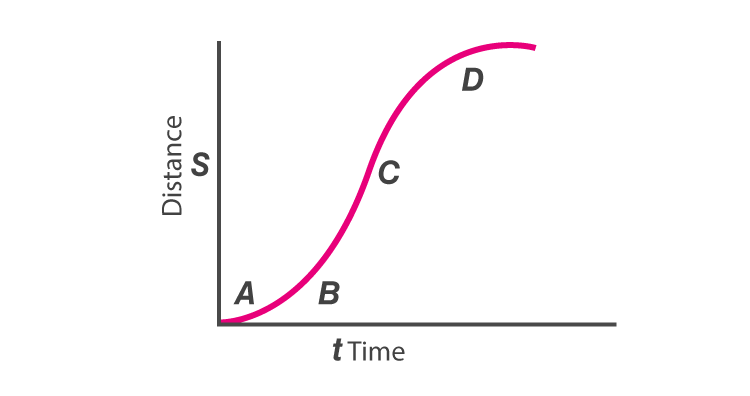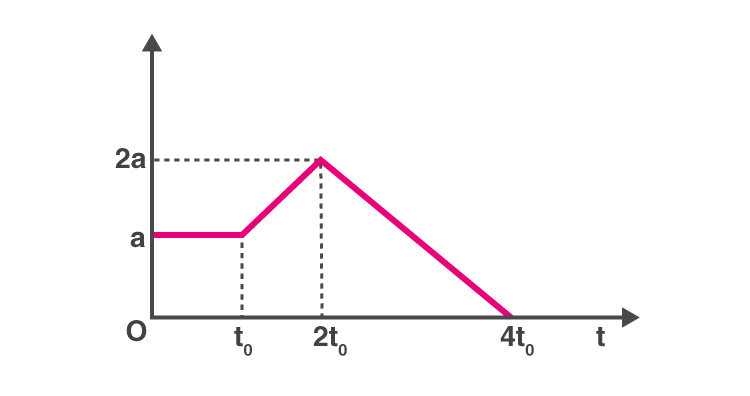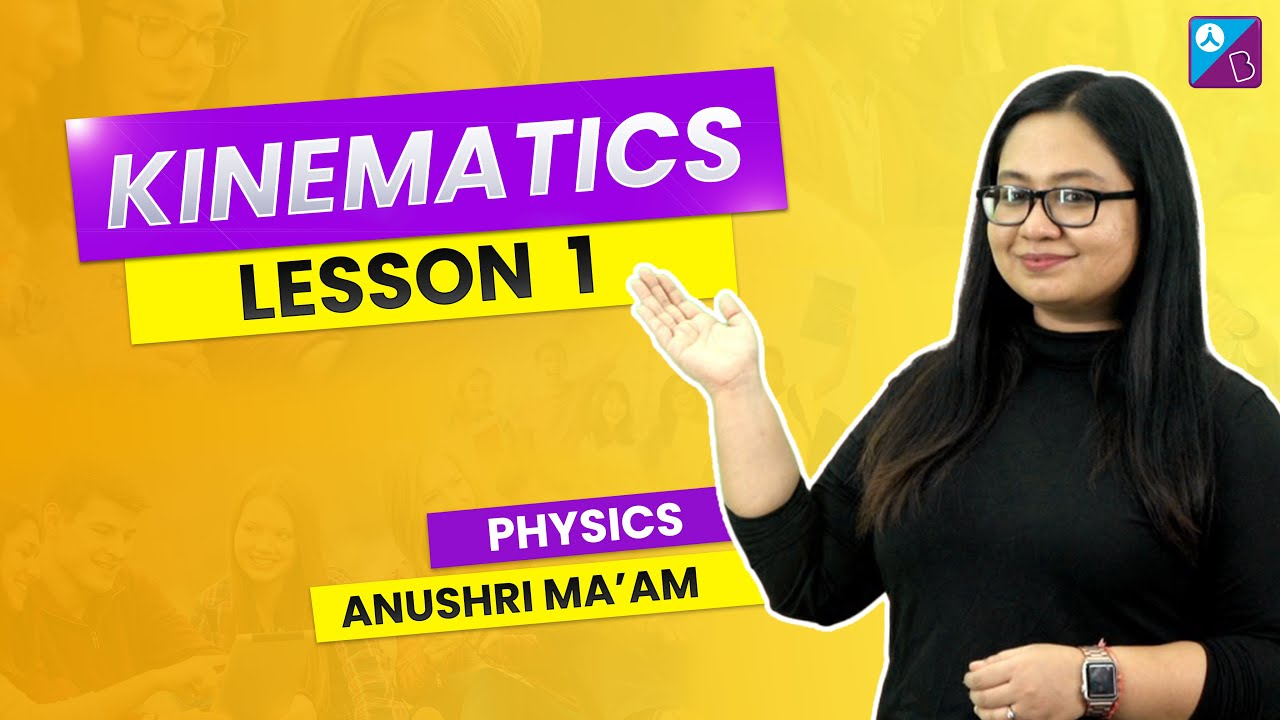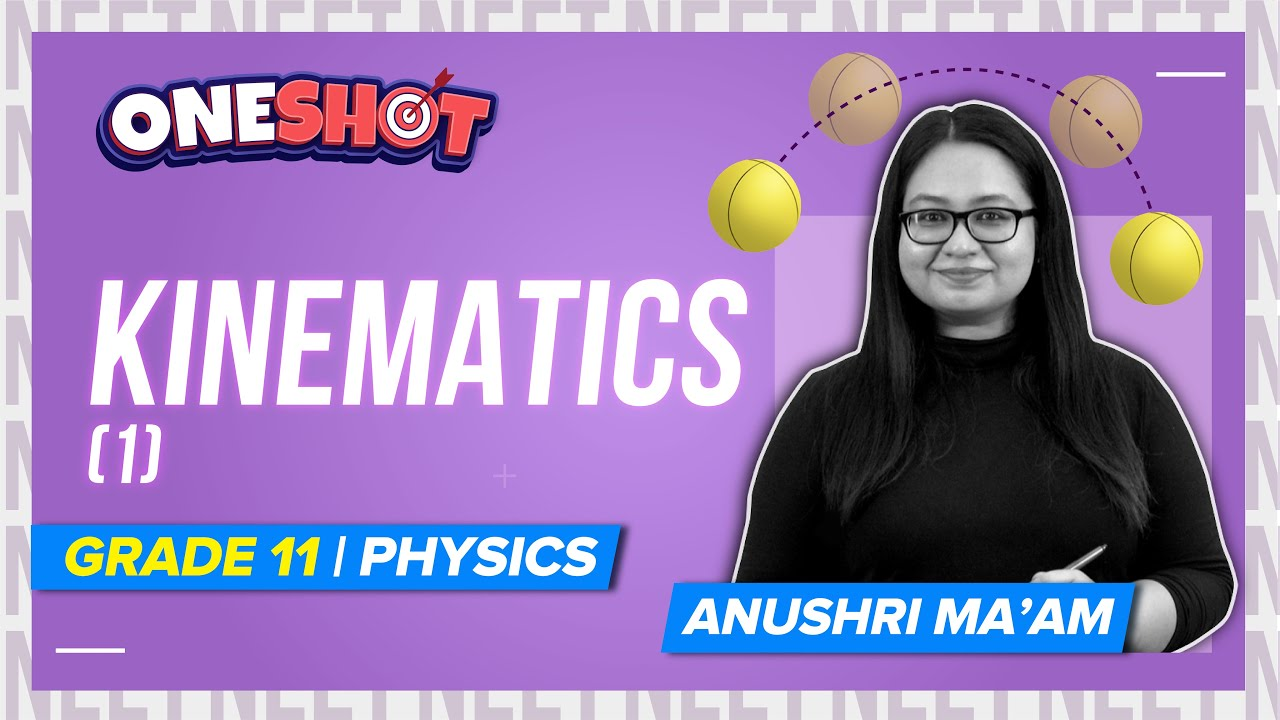# Kinematics MCQs for NEET

Kinematics is a branch of classical mechanics that deals with objects that are in motion and also other related concepts such as displacement, velocity, momentum, and acceleration. This description of the motion of an object is explained using diagrams, graphs, and equations.

Examples of kinematics are as follows:

• Train to move along the banks of the river.
• The velocity with which the ball reaches the ground when it is thrown from the fifth floor.
• The speed with which a car overtakes a bus when the roads are curved.

1. Consider two cars M and N. These cars start from the same point and travel in a straight line such that their positions are xM(t) = at + bt2 and xN(t) = ft – t2. Determine the time at which both the cars are having the same velocity

$$\begin{array}{l}(a) \frac{a-f}{1+b}\end{array}$$
$$\begin{array}{l}(b)\frac{f-a}{2(1+b)}\end{array}$$
$$\begin{array}{l}(c)\frac{a+f}{2(b-1)}\end{array}$$
$$\begin{array}{l}(d) \frac{a+f}{2(1+b)}\end{array}$$

$$\begin{array}{l}(b)\frac{f-a}{2(1+b)}\end{array}$$

2. Below is the distance-time curve traced by the particle. What is the maximum instantaneous velocity of the particle around the point(a) D

(b) B

(c) A

(d) C

3. Below is the graph of a body whose initial velocity is v0. The body travels through a straight line with acceleration as shown in the graph. Determine the maximum velocity of the body.$$\begin{array}{l}(a) v_{0}-4at_{0}\end{array}$$
$$\begin{array}{l}(b) v_{0}+4at_{0}\end{array}$$
$$\begin{array}{l}(c) v_{0}+(at_{0})\end{array}$$
$$\begin{array}{l}(d) v_{0}+\frac{9}{2}(at_{0})\end{array}$$
$$\begin{array}{l}Answer: \ (d) v_{0}+\frac{9}{2}(at_{0})\end{array}$$
$$\begin{array}{l}4. What \ will \ be \ the \ shape \ of \ the \ x\rightarrow \ y \ graph, \ if \ the \ relation \ between \ time \ and \ displacement \ of \ the \ moving \ body \ is \ t = 2αx^2 \ where α \ is \ constant.\end{array}$$

(a) Circle

(b) Straight line

(c) Parabola

(d) Hyperbola

5. Which of the following statement is true if a body moves in a semicircular track whose radius is R

(a) 2R is the displacement of the body

(b) πR is the distance travelled by the body

(c) Both (a) and (b) are correct

(d) None of the above

Answer: (c) Both (a) and (b) are correct

6. What does the area under acceleration-time graph represent for any given time interval

(a) Final velocity

(b) Distance travelled

(c) Change in the velocity in that time interval

(d) Displacement of the particle

Answer: (c) Change in the velocity in that time interval

7. When can we say that the resultant of the two vectors is maximum

(a) When the vectors are acting in the opposite direction

(b) Both the vectors are acting in the same direction

(c) When the vectors are at right angles

(d) When the vectors are parallel to each other

Answer: (b) Both the vectors are acting in the same direction

$$\begin{array}{l}8. From \ the \ below, \ select \ the \ unit \ vector \ along \ \hat{i}+\hat{j}\end{array}$$
$$\begin{array}{l}(a) \frac{\hat{i}+\hat{j}}{\sqrt{2}}\end{array}$$
$$\begin{array}{l}(b) \frac{\hat{i}+\hat{j}}{{2}}\end{array}$$
$$\begin{array}{l}(c) \frac{\hat{i}+\hat{j}}{\sqrt{4}}\end{array}$$
$$\begin{array}{l}(d) \frac{\hat{i}-\hat{j}}{\sqrt{2}}\end{array}$$

$$\begin{array}{l}(a) \frac{\hat{i}+\hat{j}}{\sqrt{2}}\end{array}$$

9. A particle with radius R is moving in a circular path with constant speed. The time period of the particle is T.

$$\begin{array}{l}Calculate \ the \ time \ for \ the \ following \ after \ t=\ \frac{T}{6}. \end{array}$$

What is the average velocity of the particle?

$$\begin{array}{l}(a) \frac{3 R}{T}\end{array}$$
$$\begin{array}{l}(b)\frac{4 R}{T}\end{array}$$
$$\begin{array}{l}(c)\frac{6 R}{T}\end{array}$$
$$\begin{array}{l}(d)\frac{2 R}{T}\end{array}$$
$$\begin{array}{l}Answer: \ (c)\frac{6 R}{T}\end{array}$$

### Recommended Video:Don’t miss these NEET Physics MCQs: